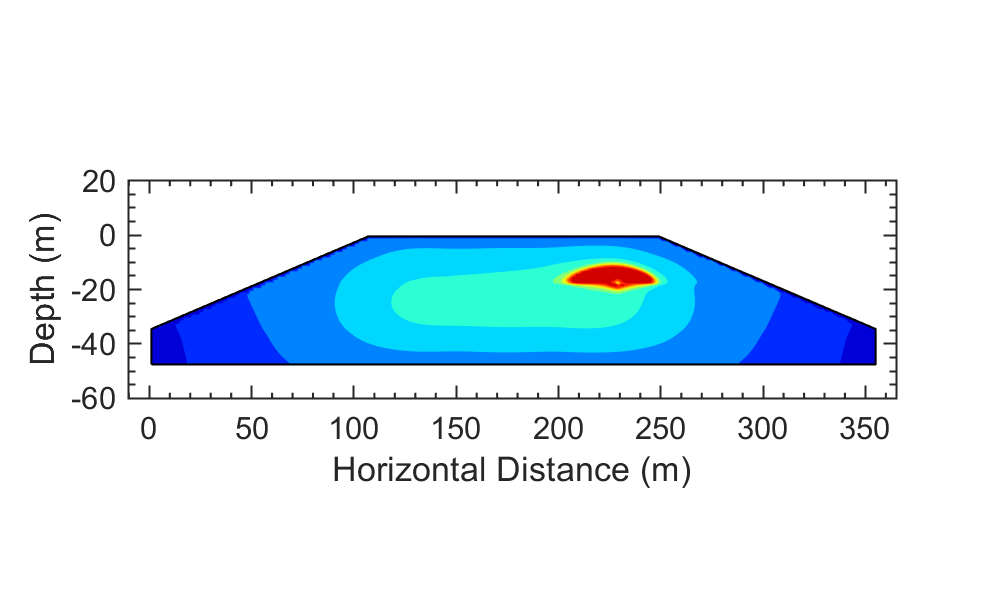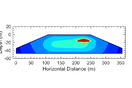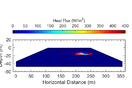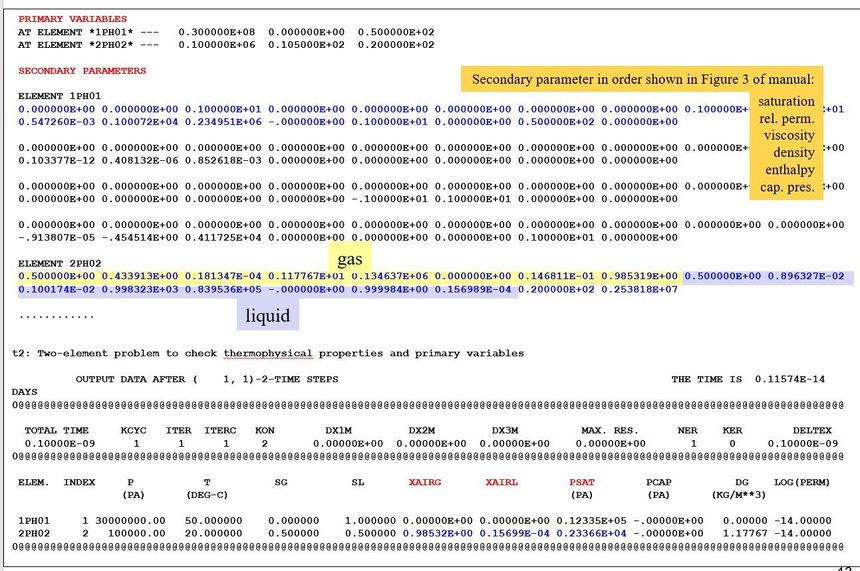0# Calculating Total Heat Generated in an AreaDear all,

I am working with TOUGH2 Landfill Bioreactor Model (T2LBM) to simulate a biological reaction in my domain which stimulates by injecting oxygen. The biological reaction generates heat flux after stimulation and causes the temperature to increase (please note the two pictures, one is temperature and the other is heat flux rate). I used the GENER block to inject oxygen into one grid block, and the oxygen spreads out to the adjacent grid blocks as well. Therefore, the biological reaction stimulates not only in the injection block but also in a few other blocks. I am trying to find a way to calculate the total heat generated in the impacted domain, more specifically, the grid blocks that receive oxygen. Please let me know your recommendations.

Best regards,

Alborz

5replies Oldest first
• Oldest first
• Popular•This is kind of an ad hoc approach, but if pressure changes are small, so little heat is being created by mechanical work, then you might be able to approximate the heat content of each grid block as the product of the temperature change and the heat capacity and the volume of the grid block.  You will need the temperature change from initial temperature (DeltaT), grid-block volume (Vol), the porosity (por) to determine how much rock and how much fluid you have, the phase saturations (Sl, Sg), and the volumetric heat capacity (product of density (kg/m3) and specific heat (J/(kg K)) ) of each phase and rock.  I am not sure about what T2LBM prints out, but it may not include fluid heat capacities, so you will have to look them up elsewhere.

Heat = [(1-por)*dens_rock*spec_heat_rock + por*(Sl*dens_liq*spec_heat_liq + Sg*dens_gas*spec_heat_gas)]*DeltaT*Vol.

Like
•Dear Christine,

Before trying to go with your approach, I would like to share the T2LBM output and explain what method I am using to calculate the heat so maybe you can correct me if I'm wrong.

Considering we want to know how much heat is generated/accumulated in a single cell. We have 5 cells where C is in the center, A and E are left and right to C, respectively; B and D are above and below C, respectively. The output file is showing heat flux between every two cells.

A-C  450 W/m2

B-C  70 W/m2

C-E  490 W/m2

C-D  -10 W/m2

My approach is to first get an average heat flux for C for x and y-directions:

Heat flux y-direction = (70-10)/2 = 30 W/m2

Heat flux x-direction = (490+450)/2 = 470 W/m2

Then the total heat generated/accumulated in Cell C = (30^2 + 470^2)^0.5 = 471 W/m2

Same approach for any other cell in the area of interest. Is this false?

Thanks,

Alborz

Like
•Dear Alborz,  Sorry for the late reply - I forgot to check the Forum for a few days.

My first thought when answering your original query was to try to use heat flux, since T2LBM  shows heat flux as part of the output.   But I don't think that heat flux tells the whole story, unless the system is at a steady state with temperature not changing anywhere.  If that is the case, you could add up all the fluxes in and out - but I don't think you should do the square root of the squares. In general, if temperatures are changing, you need to do a complete heat balance that combines flux and temperature change in a grid block.  Of course, this is what TOUGH does as part of its calculations, it just does not print it out.  The best solution to your problem would be modify T2LBM to print out the information you want but that would require you to have the source code and be at least somewhat familiar with FORTRAN programming.  Another option would be to set MOP(5)=9.  This will print out all the secondary parameters for every grid block every time step.  You should only do this for a simulation consisting of one or two time steps, otherwise the file will get too huge.  The secondary parameters are shown in Figure 3 of the TOUGH2 User's Guide.  The figure below shows a screen-shot from the TOUGH Beginner's Course, where this output is discussed.  For each grid block there are 4 sets of parameters, you want to look at the first one.  It shows parameters for liquid phase, then gas phase.  The 5th entry of each is specific enthalpy in J/kg.  This replaces (C delta T) in my formula above.  Sorry this got so long.  Hope it helps.  ChristineLike
Like Follow